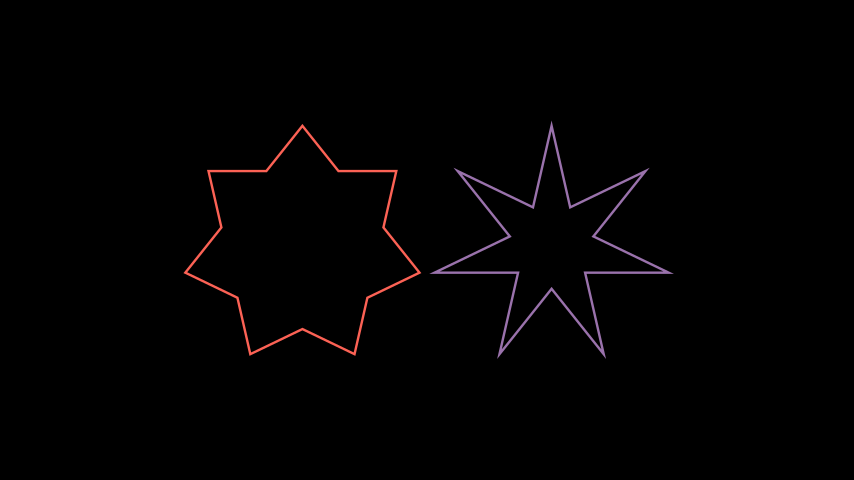# Star¶

Qualified name: `manim.mobject.geometry.Star`

A regular polygram without the intersecting lines.

Parameters
• n (int) – How many points on the `Star`.

• outer_radius (float) – The radius of the circle that the outer vertices are placed on.

The radius of the circle that the inner vertices are placed on.

If unspecified, the inner radius will be calculated such that the edges of the `Star` perfectly follow the edges of its `RegularPolygram` counterpart.

• density (int) –

The density of the `Star`. Only used if `inner_radius` is unspecified.

See `RegularPolygram` for more information.

• start_angle (Optional[float]) – The angle the vertices start at; the rotation of the `Star`.

• kwargs – Forwardeds to the parent constructor.

Raises

ValueError – If `inner_radius` is unspecified and `density` is not in the range `[1, n/2)`.

Examples

Example: StarExample```from manim import *

class StarExample(Scene):
def construct(self):

self.play(Create(star), run_time=3)
```

Example: DifferentDensitiesExample```from manim import *

class DifferentDensitiesExample(Scene):
def construct(self):
density_2 = Star(7, outer_radius=2, density=2, color=RED)
density_3 = Star(7, outer_radius=2, density=3, color=PURPLE)

 `animate` Used to animate the application of a method. `animation_overrides` `depth` The depth of the mobject. `height` The height of the mobject. `width` The width of the mobject.